﻿ 仿人机器人步态平衡泛化模型的建立与仿真
«上一篇文章快速检索 高级检索

 智能系统学报2020, Vol. 15Issue (3): 537-545  DOI: 10.11992/tis.2018100170

### 引用本文YU Jianjun, LI Chen, ZUO Guoyu, et al. Modeling and simulation of humanoid robot gait balance generalization[J]. CAAI Transactions on Intelligent Systems, 2020, 15(3): 537-545. DOI: 10.11992/tis.201810017.### 文章历史

Modeling and simulation of humanoid robot gait balance generalization
YU Jianjun, LI Chen , ZUO Guoyu , RUAN Xiaogang , WANG Yang
Department of Information, Beijing University of Technology, Beijing 100124, China
Abstract: The problem of robot walking instability can be solved by calculating the zero-moment point (ZMP) through human body teaching and correction by the compensation of joint angles; however, problems such as high algorithm complexity still exist. This paper proposes a method that combines human teaching with machine learning. The gait balance generalization model of a robot is established based on the support vector regression algorithm. The joint angle of human teaching and ZMP information are inputted into the model; then, we get the joint angle compensated by stability, and the robot is driven to complete the walking action. The parameters of the whale optimization algorithm (WOA) model are introduced to make the model obtain the optimal generalization effect and improve the performance of the gait balance model. Under the Webots simulation platform, the NAO robot is driven by the compensated joint angle of the model output. The action is natural and stable, and the algorithm complexity is low, which verifies the feasibility of the method.
Key words: humanoid robot    support vector regression    gait balance generalization model    whale optimization algorithm    ZMP information    algorithm complexity    NAO robot    machine learning

1 基于SVR的机器人步态平衡泛化模型的构建

1.1 支持向量回归算法

 ${{D = }}\left\{ {\left( {{{{x}}_1},\;\;{{{y}}_1}} \right),\left( {{{{x}}_2},\;\;{{{y}}_2}} \right), \cdots ,\left( {{{{x}}_i},\;\;{{{y}}_{{i}}}} \right),\;\;{{i = }}1,2, \cdots ,\;l} \right\}$

 ${{f}}\left( {{x}} \right) = \omega {{\cdot}}\varPhi \left( {{x}} \right){{ + b}}$

 $\left\{ \begin{array}{l} \mathop {{\rm{min}}}\limits_{\left( {\omega ,b,{\xi _i},{\xi _i}^*} \right)} \dfrac{1}{2}{\left\| \omega \right\|^2} + C\displaystyle\sum\limits_{i = 1}^l {\left( {{\xi _i} + {\xi _i}^*} \right)} \\ {\rm{s}}{\rm{.t}}{\rm{.\; }}{y_i} - \omega \varPhi \left( x \right) - b \leqslant \varepsilon + {\xi _i}\\ {\rm{ }}\omega \varPhi \left( x \right){\rm{ + }}b \leqslant \varepsilon + {\xi _i}^*\\ {\rm{ }}{\xi _i},{\xi _i}^* \geqslant 0 \end{array} \right.$ (1)

 $\left\{ \begin{array}{l} \mathop {{\rm{max}}}\limits_{\left( {{\alpha _i},{\alpha _i}^*} \right)} \displaystyle\sum\limits_{i = 1}^l {{\alpha _i}\left( {y - \varepsilon } \right)} - \displaystyle\sum\limits_{i = 1}^l {{\alpha _i}^*\left( {y + \varepsilon } \right)} +\\ {\rm{ }}\;\;\;\;\;\;\;\; \displaystyle\sum\limits_{i,j = 1}^l {\left( {{\alpha _j} - {\alpha _j}^*} \right)} \left( {{\alpha _i} - {\alpha _i}^*} \right)K\left( {{x_i},{x_j}} \right)\\ {\rm{s}}{\rm{.t}}{\rm{.\; 0}} \leqslant {\alpha _i} \leqslant C,{\rm{0}} \leqslant {\alpha _i}^* \leqslant C,i = 1,2, \cdots ,l\\ \displaystyle\sum\limits_{i = 1}^l {\left( {{\alpha _i} - {\alpha _i}^*} \right) = 0} \end{array} \right.$ (2)

 $f(x)=\sum _{i=1}^{l}\left({\alpha }_{i}-{\alpha }_{i}^{*}\right)K\left({x}_{i},{x}_{j}\right)+b$ (3)

1.2 仿人机器人步态平衡泛化模型的构建

 ${{p}} = c - \frac{h}{g}{{\ddot c}}$ (4)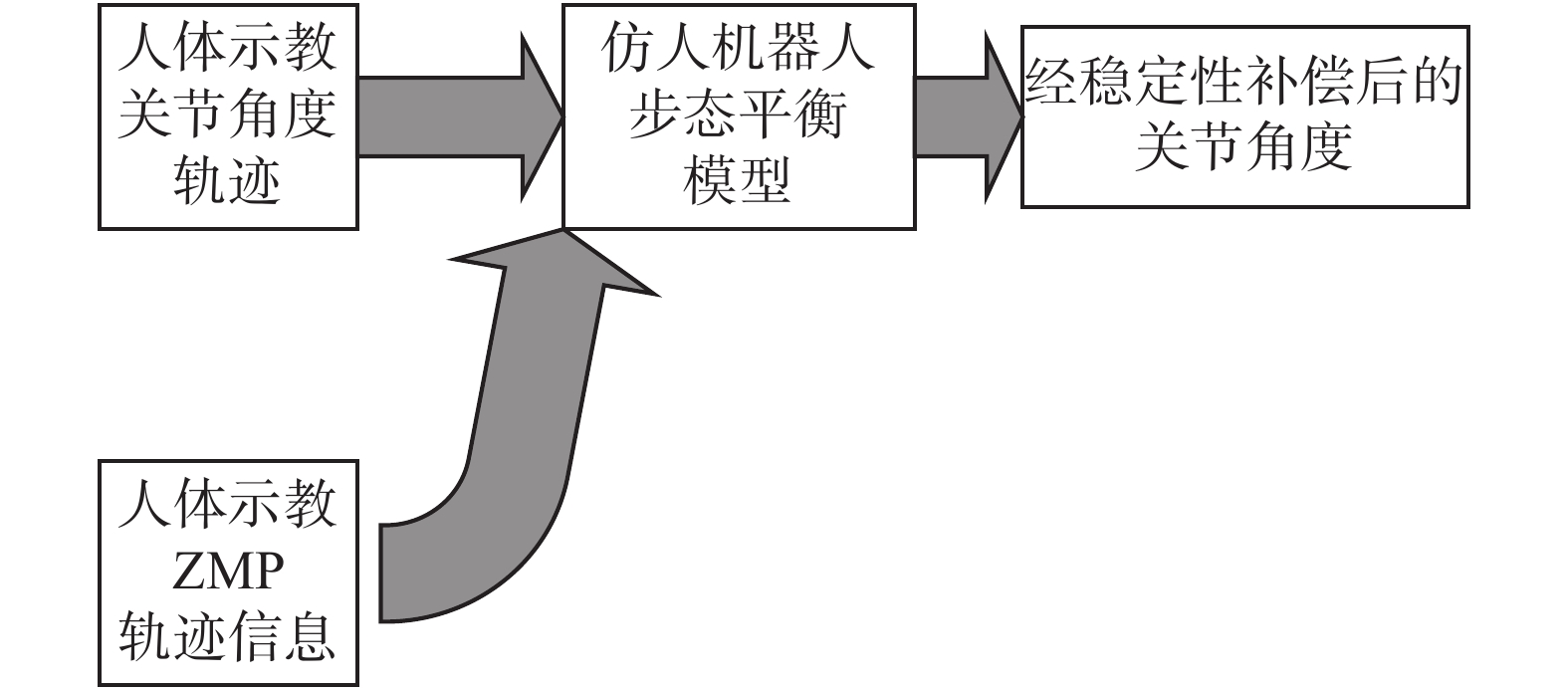Download: 图 1 机器人步态平衡泛化模型结构 Fig. 1 Robot gait balance generalization model structure

2 基于WOA的仿人机器人步态平衡泛化模型的参数寻优

2.1 鲸鱼优化算法

 $\left\{ \begin{array}{l} {{D}} = \left| {{{C}} \cdot {{{x}}^{{*}}}\left( t \right) - {{x}}\left( t \right)} \right| \\ {{x}}\left( {t + 1} \right) = {{{x}}^{{*}}}\left( t \right) - {{A}} \cdot {{D}} \\ {{A}} = 2{{a}} \cdot {{r}} - {{a}} \\ {{C}} = 2 \cdot {{r}} \\ \end{array} \right.$ (5)

 ${{x}}\left( {{\rm{t}} + 1} \right) = \left\{ {\begin{array}{*{20}{l}} {{{{x}}^{{*}}}\left( t \right) - {{A}} \cdot {{D}},\quad{{p}} < 0.5}\\ {{{D}} \cdot {{\rm{e}}^{{{bl}}}} \cdot {\rm{cos}}\left( {2{{{\text{π}} l}}} \right){{ + }}{{{x}}^{{*}}}\left( t \right),\quad{{p}} \geqslant 0.5} \end{array}} \right.$ (6)

 $\left\{ {\begin{array}{*{20}{l}} {{{D}} = \left| {{{C}} \cdot {{{x}}_{{\rm{rand}}}} - {{x}}\left( t \right)} \right|} \\ {{{x}}\left( {t + 1} \right) = {{{x}}_{{\rm{rand}}}} - {{A}} \cdot {{D}}} \end{array}} \right.$ (7)

2.2 WOA与传统算法对SVR非线性回归模型参数优化的能力比较表 1 3种算法对SVR非线性回归模型寻优效果比较 Tab.1 Comparison of effects of three algorithms for SVR nonlinear regression model

2.3 基于WOA优化算法的机器人步态平衡泛化模型的参数优化

1)确定3个参数的寻优范围分别为 $p$ (0.005,0.3)、 $C$ (0.05,3)、 $\varepsilon$ (1,4)，构成WOA的寻优空间。

2)构建WOA的适应性函数。针对 $\varepsilon -$ SVR算法的训练参数的优化问题，选择MSE函数作为适应性函数。

3)使用WOA迭代寻优，在寻优空间内确定一组Cp $\varepsilon$ ，使训练的MSE值最小。

3 仿真实验设计与结果分析

1)使用Kinect2.0对人体步行动作进行数据采集。Kinect2.0是一款能对人体的骨骼点信息进行跟踪，记录体感的采集器，它能对人体的25个骨骼点进行跟踪，并记录其三维坐标(xyz)。

2)在MATLAB中，对人体示教数据进行处理并构建平衡泛化模型，具体步骤为：运用空间向量法进行人体关节角度的计算；通过人体在步行运动双脚的高度选取支撑脚，以此脚底部的骨骼点作为ZMP信息提取，并进行数据预处理(归一化)；SVR非线性模型构建；利用模仿学习计算平衡性补偿角的方式到经补偿后的机器人关节角度构成训练集和测试集；WOA参数寻优；步态平衡泛化模型训练和泛化。

3)在WEBOTS的仿真平台上，使用模型泛化补偿后的关节角驱动NAO机器人，观察其运动效果。其中，NAO机器人是由Aldebaran Robotics公司研发的有着13个关节25个自由度的仿人机器人，适合作为仿人机器人算法的研究平台。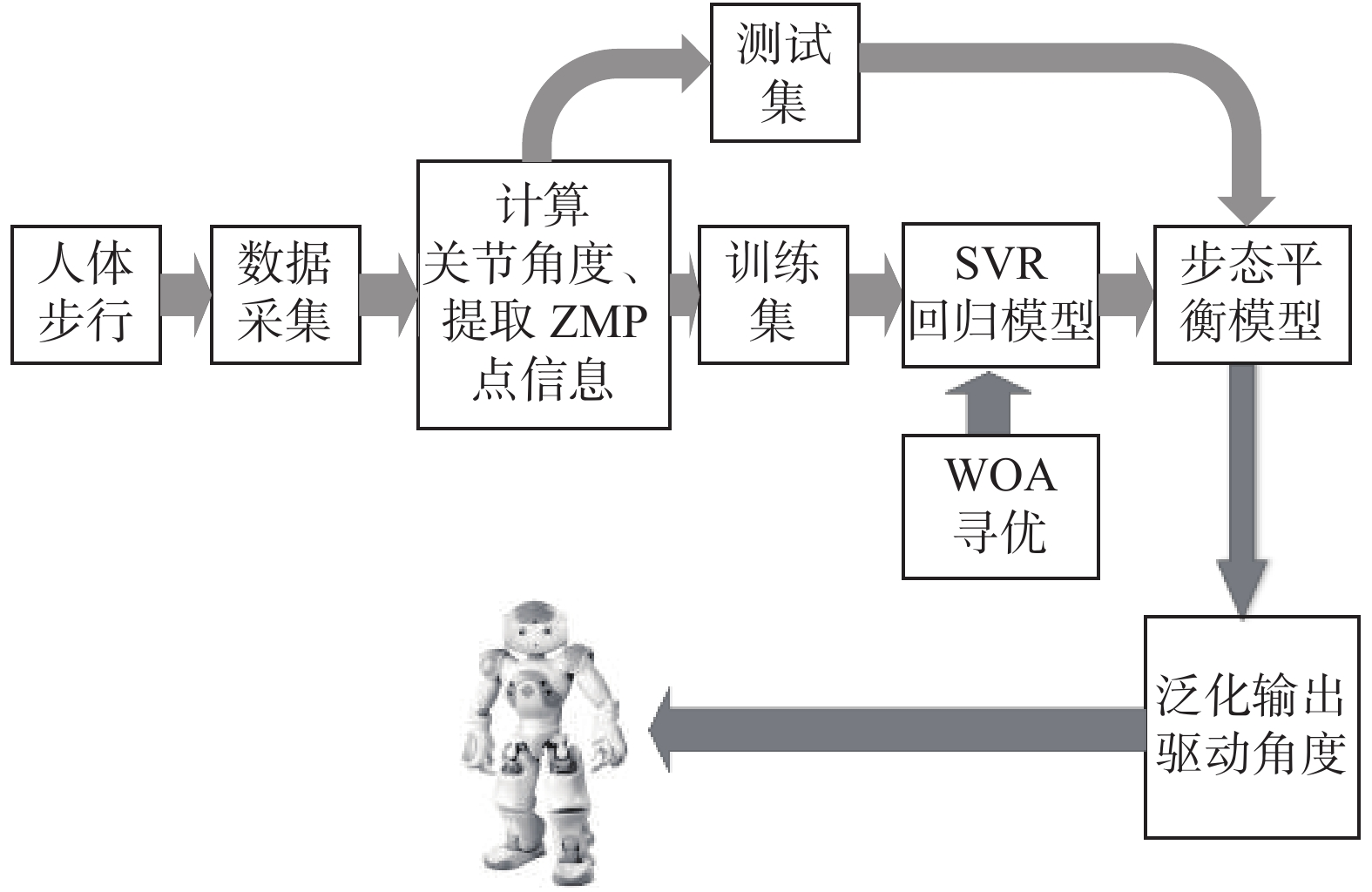Download: 图 2 仿真流程图 Fig. 2 Simulation flowchart
3.1 仿人机器人步态平衡模型的训练

Kinect2.0采集一组人体行走数据，共177个数据点(一个步态周期)，前130个作为训练集，后47个作为测试集。由于仿人机器人的关节较多，在其步行运动过程中，通过调整髋关节和踝关节8个关节角度便可以实现稳态步行。因此以左腿为例，对左腿髋关节和踝关节的4个关节角度进行步态平衡模型的构建和训练。机器人踝关节的平衡泛化模型结构如图3所示。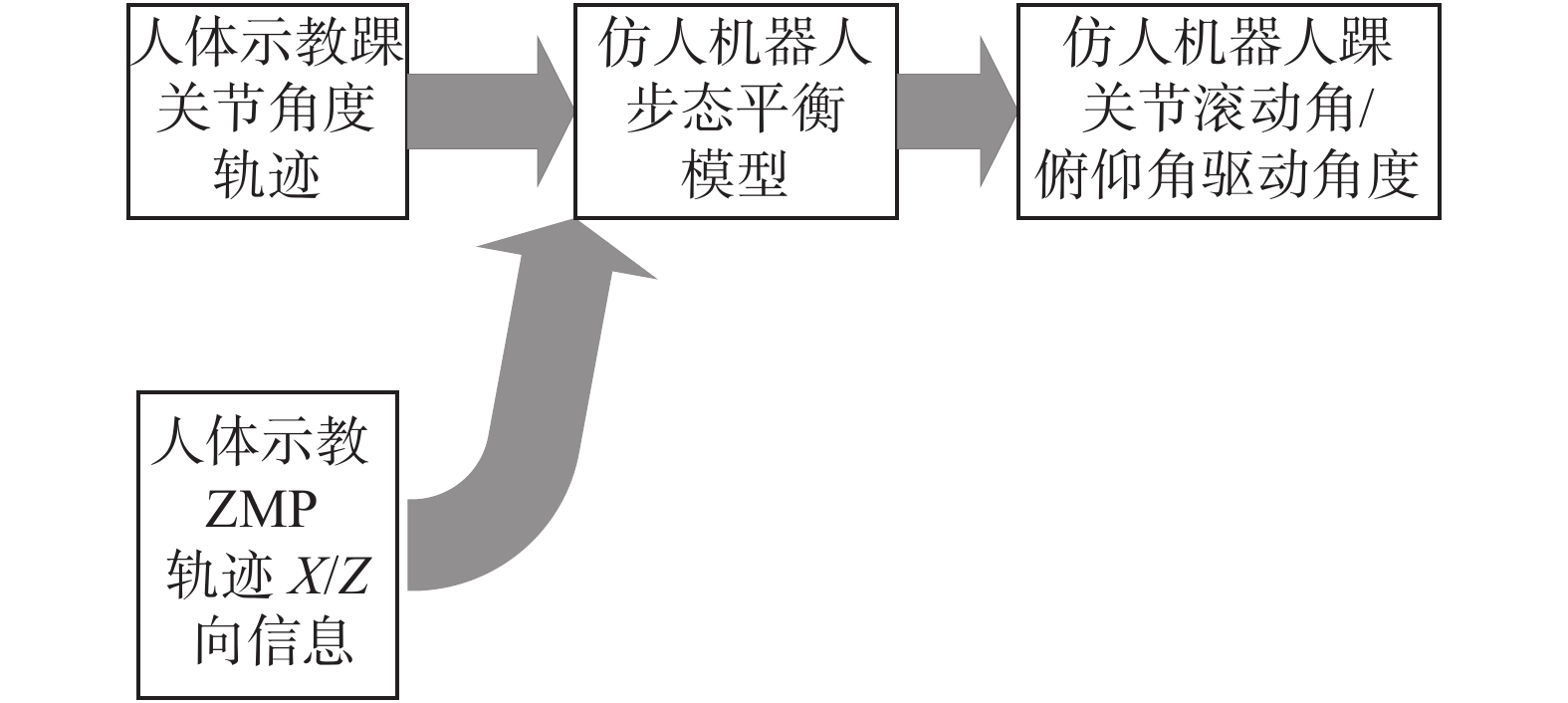Download: 图 3 踝关节步态平衡泛化模型结构图 Fig. 3 Ankle joint gait balance generalization model structure diagram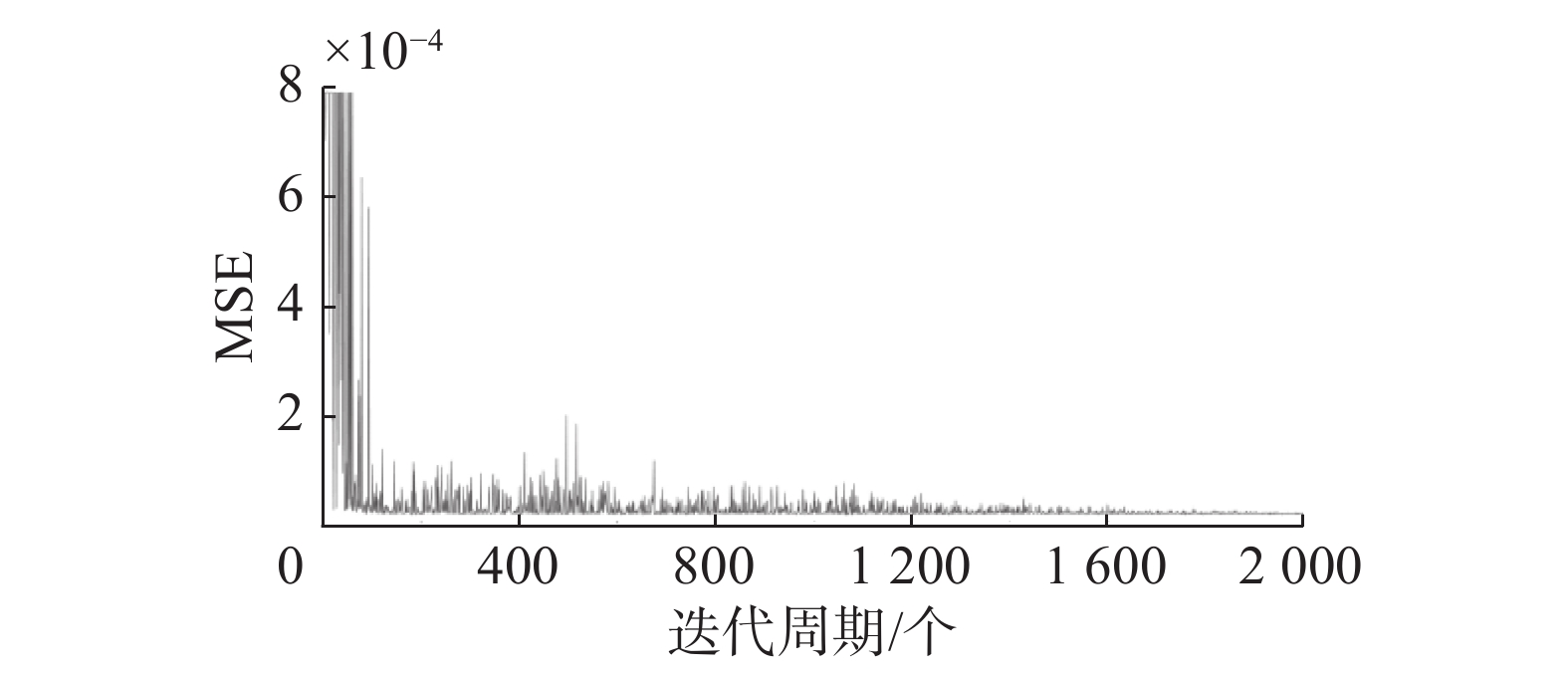Download: 图 4 WOA寻优MSE变化曲线图 Fig. 4 WOA optimization MSE curve表 2 仿人机器人步态平衡泛化模型的训练参数及训练性能指标 Tab.2 Training parameters and training performance indicators of humanoid robot gait balance generalization model

3.2 仿人机器人步态平衡模型的泛化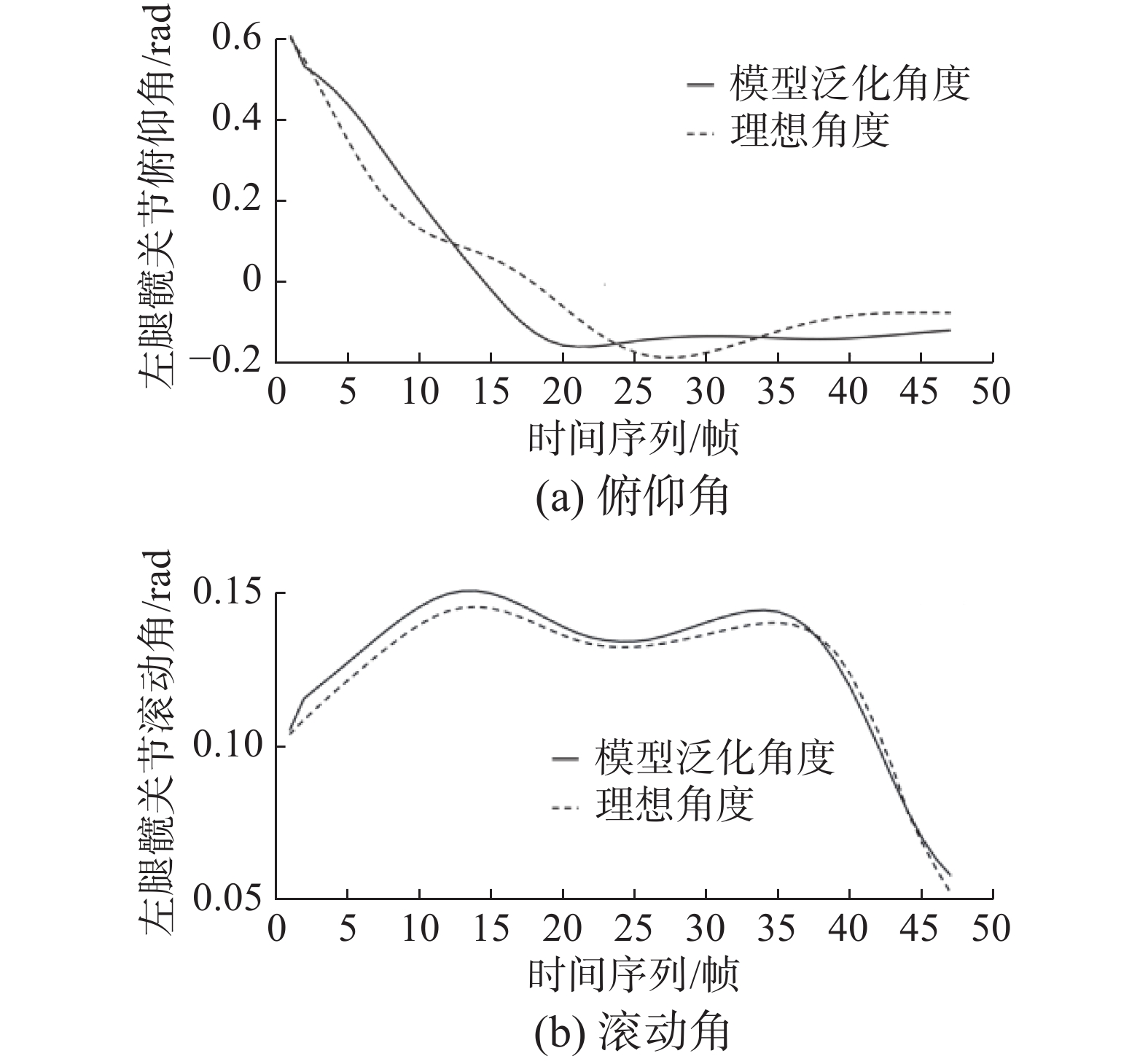Download: 图 5 髋关节泛化结果 Fig. 5 Hip generalization results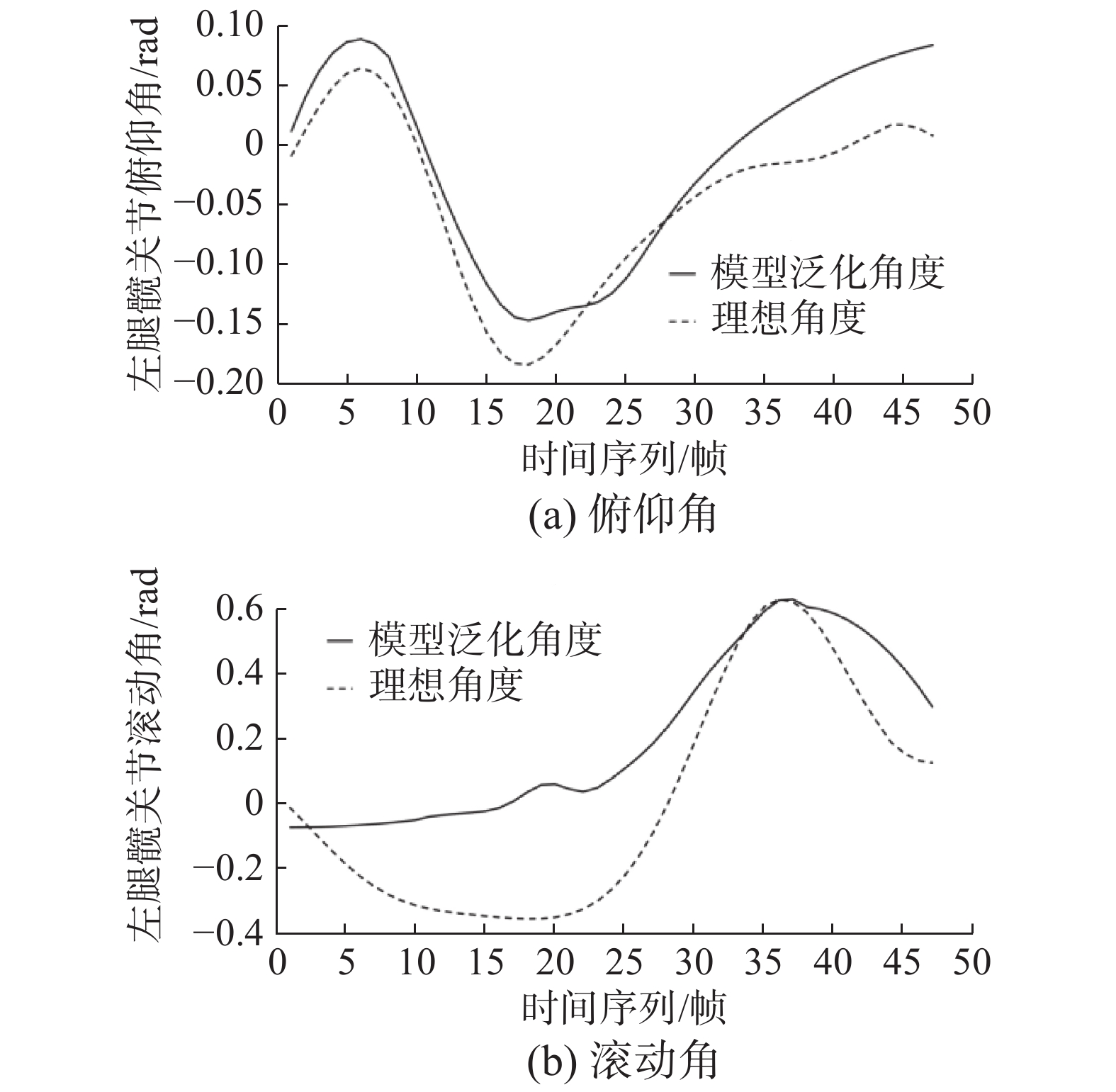Download: 图 6 踝关节泛化结果 Fig. 6 Ankle generalization results表 3 仿人机器人步态平衡泛化模型在同一步长、步态周期下的泛化性能指标 Tab.3 Generalized performance indicators of humanoid robot gait balance generalization model under the same step and gait cycle

 ${\delta _{{c}}} = {{J}}{\delta _\alpha }$ (9)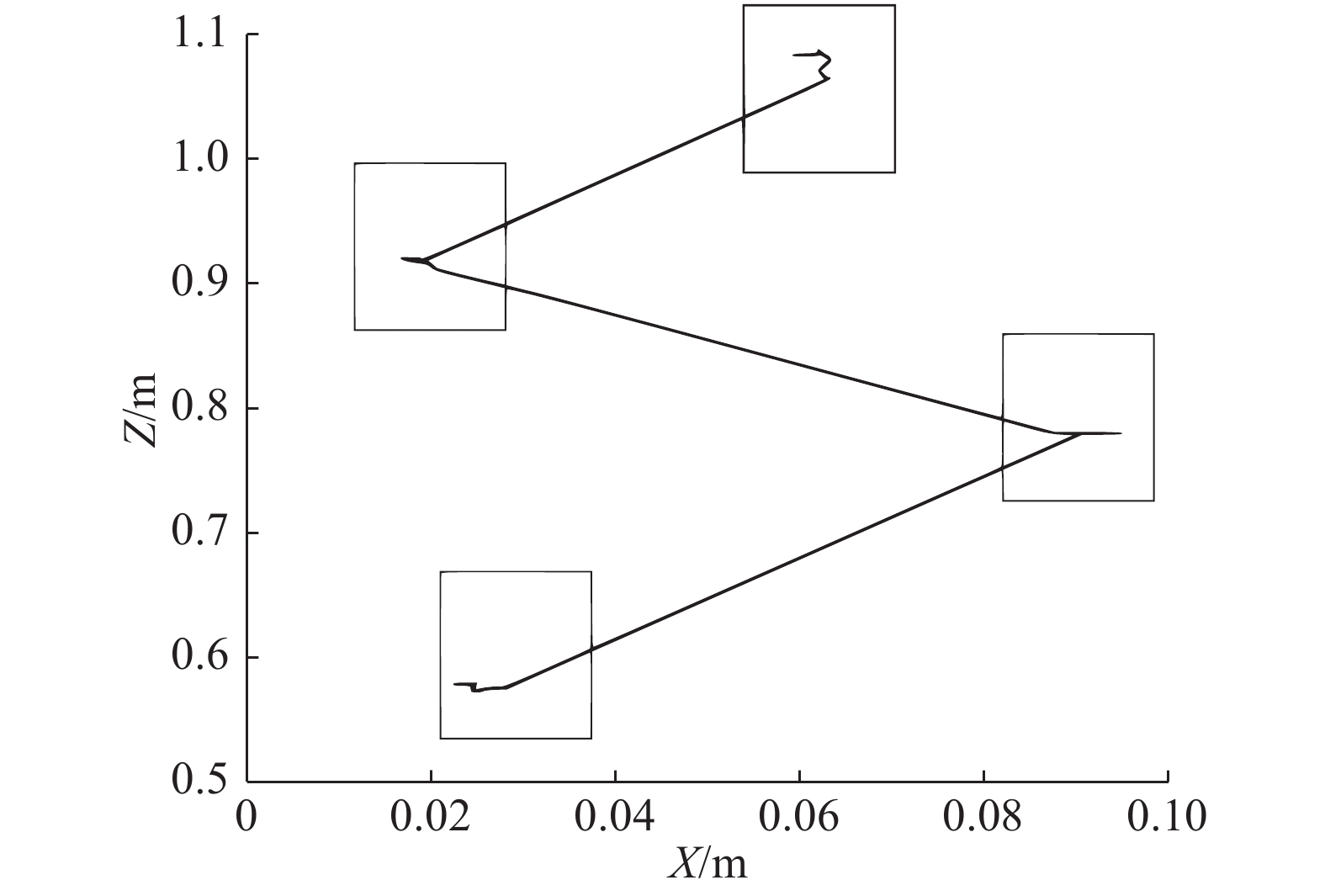Download: 图 7 同一步长、步态周期下在泛化驱动角度下机器人ZMP轨迹 Fig. 7 Robot ZMP trajectory at generalized driving angle under the same step and gait cycle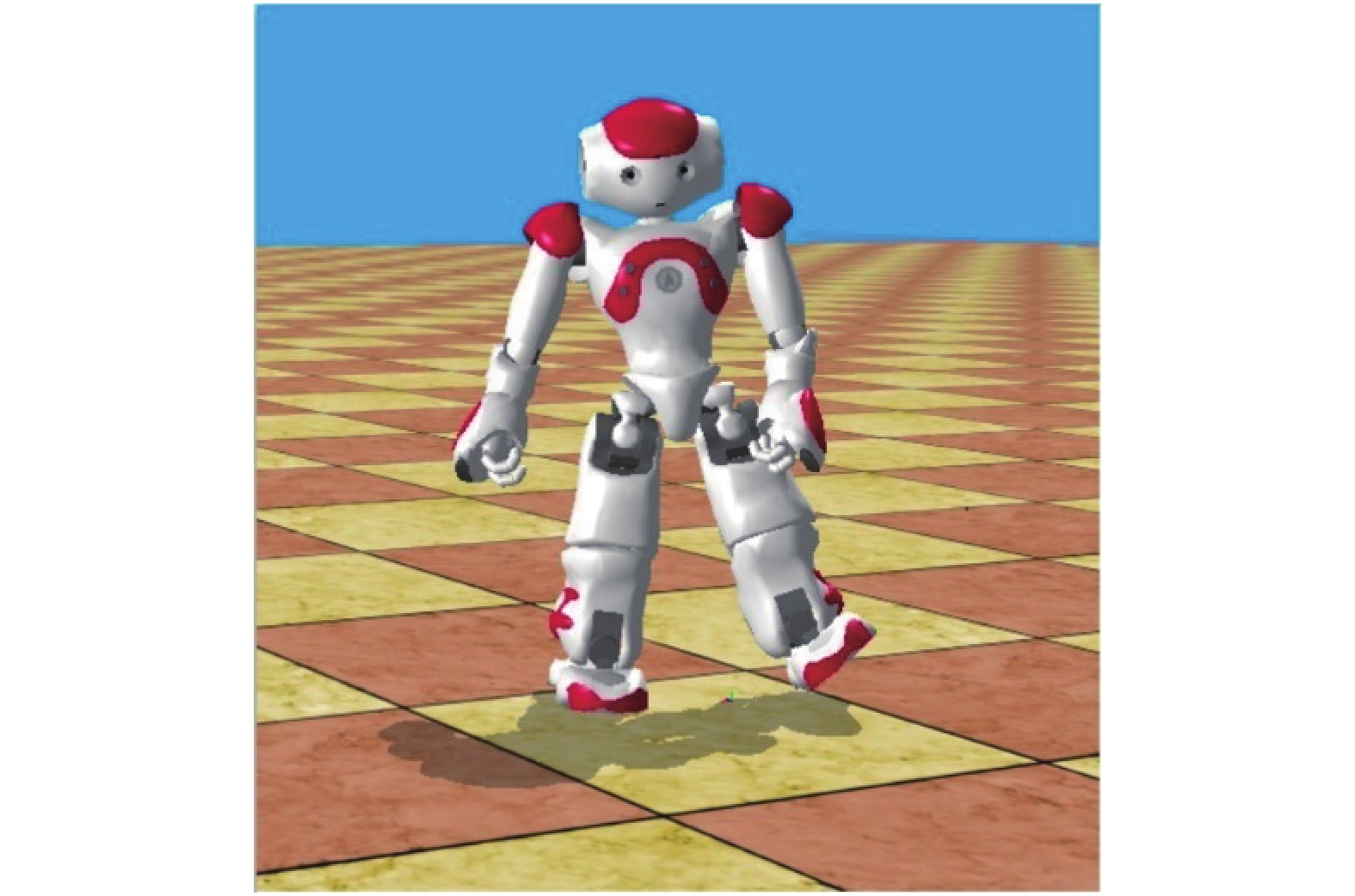Download: 图 8 模型泛化角度值在WEBOTS仿真平台上的稳定步行 Fig. 8 Model generalized angle values for stable walking on the WEBOTS simulation platform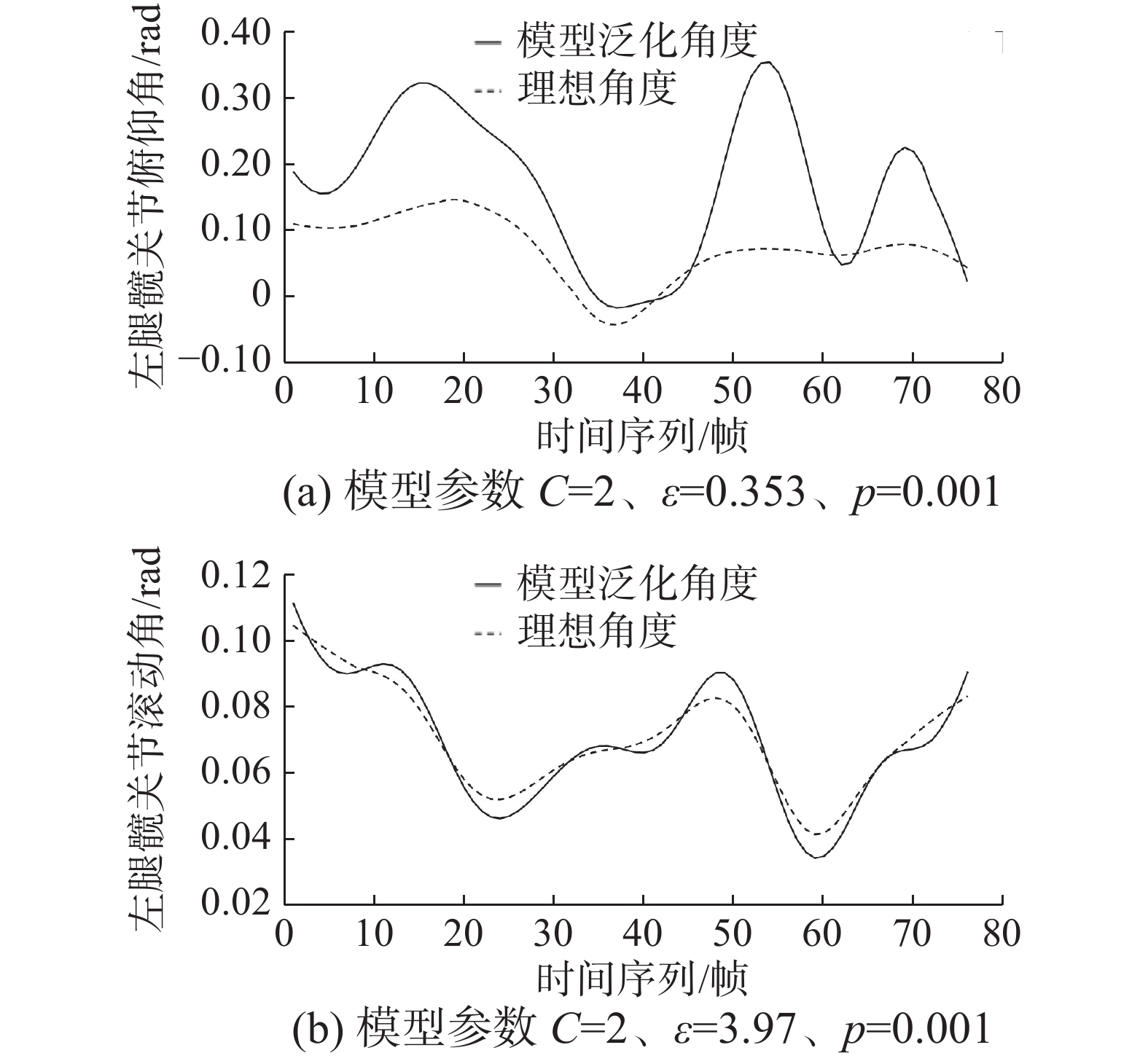Download: 图 9 不同步长、步态周期下左腿髋关节泛化结果 Fig. 9 Generalization of left hip pitch under different gait long and gait cycles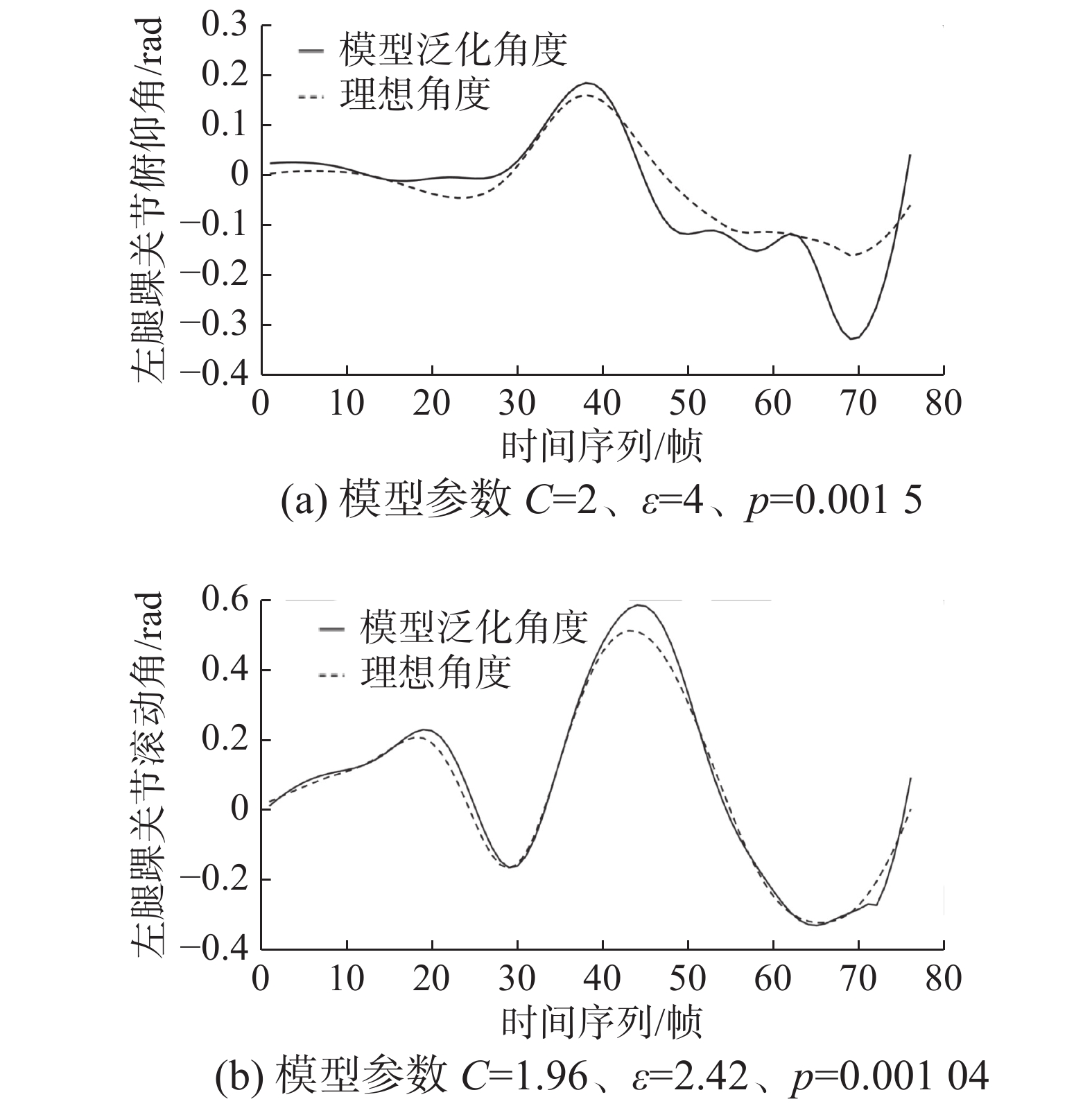Download: 图 10 不同步长、步态周期下左脚踝关节泛化果 Fig. 10 Generalization of left ankle roll under non-synchronized and gait cycles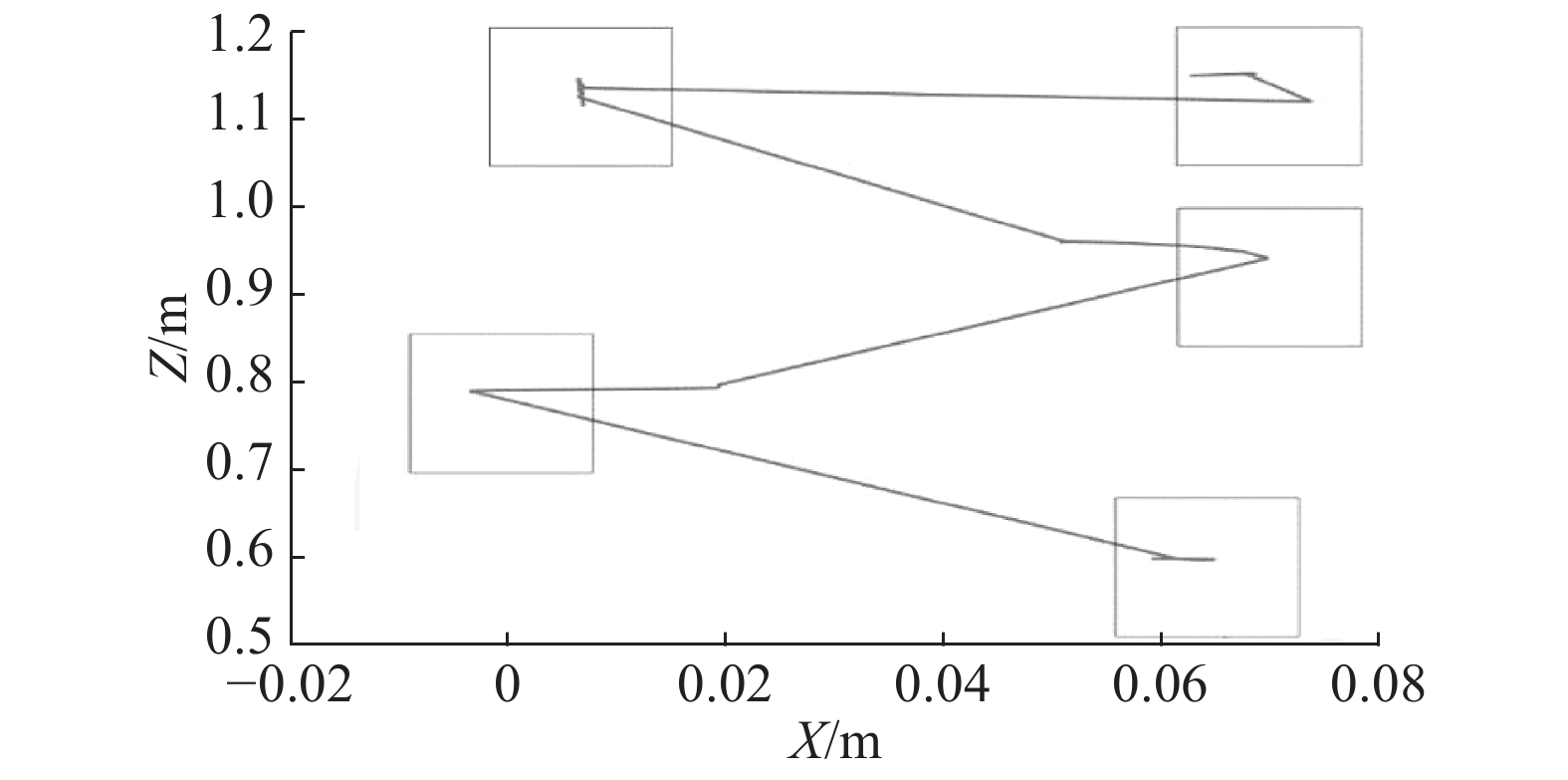Download: 图 11 不同步长、步态周期下的机器人ZMP轨迹 Fig. 11 Robot ZMP trajectory under different gait long and gait cycle表 4 不同步长、步态周期下的泛化性能指标 Tab.4 Generalized performance indicators of unsynchronized and long gait cycles

1)由于Kinect是一款非专业的体感采集器，其测量范围有一定的限制，太远或太近都会使测量误差过大，无法进行一组两个以上步行动作的采集。同时结合同一步长、步态周期下，泛化的起始点与理想的起始点有一些偏差，导致在循环动作时机器人会有一定不自然动作，甚至会导致机器人不稳定、摔倒。因此，在保证机器人稳定的基础上，步态周期间的平滑切换(连续步态周期的关节角度时间序列预测)是下一阶段工作的重点之一[17-19]

2)在不同步长、步态周期下的模型泛化效果与同一步长、步态周期下的相比不够理想。因此，进一步优化模型的算法和选择训练模型数据是构建针对仿人机器人不同组步行数据下的平衡泛化模型和构建步态循环模型的需要解决的问题。

4 结束语

  WANG Fei, WANG Yaning, WEN Shiguang, et al. Nao humanoid robot gait planning based on the linear inverted pendulum[C]//Proceedings of 2012 24th Chinese Control and Decision Conference. Taiyuan, China, 2012: 986−990. (0)  DONG Enzeng, WANG Dandan, CHEN Chao, et al. Realization of biped robot gait planning based on NAO robot development platform[C]//Proceedings of 2016 IEEE International Conference on Mechatronics and Automation. Harbin, China, 2016: 1073−1077. (0)  ZHANG Ying, LI Shuanghong, HAN Boyu, et al. Research on gait planning and inverse kinematics solving of biped walking robots[C]//Proceedings of 2015 8th International Symposium on Computational Intelligence and Design. Hangzhou, China, 2015: 629−632. (0)  KHAZENIFARD A, BAHRAMI F, ANDANI M E, et al. An energy efficient gait trajectory planning algorithm for a seven linked biped robot using movement elements[C]//Proceedings of 2015 23rd Iranian Conference on Electrical Engineering. Tehran, Iran, 2015: 1006−1011. (0)  付根平, 杨宜民, 黄春林. 基于粒子群优化算法的双足机器人步态优化[J]. 华中科技大学学报(自然科学版), 2011, 39(S2): 355-358. FU Genping, YANG Yimin, HUANG Chunlin. Walking pattern optimization based on particle swarm optimization for biped robot[J]. Journal of Huazhong University of Science and Technology (nature science edition), 2011, 39(S2): 355-358. (0)  YAMAGUCHI A, HYON S H, OGASAWARA T. Reinforcement learning for balancer embedded humanoid locomotion[C]//Proceedings of 2010 10th IEEE-RAS International Conference on Humanoid Robots. Nashville, USA, 2010: 308−313. (0)  KANG Zhongrun, DENG Muqing, WANG Cong. Frontal-view human gait recognition based on kinect Features and deterministic learning[C]//Proceedings of 2017 36th Chinese Control Conference. Dalian, China, 2017: 10834−10839. (0)  SELEEM I A, ASSAL S F M. Sliding mode control of underactuated five-link biped robot for climbing stairs based on real human data[C]//Proceedings of 2017 IEEE International Conference on Industrial Technology. Toronto, Canada, 2017: 878−883. (0)  赵姝颖, 徐文杰, 郑雪林, 等. 基于体感的机器人展示系统研究与开发[J]. 机器人技术与应用, 2012(6): 54-57. ZHAO Shuying, XU Wenjie, ZHENG Xuelin, et al. Research and development of display system of Nao humanoid robot based on posture perception[J]. Robot technique and application, 2012(6): 54-57. DOI:10.3969/j.issn.1004-6437.2012.06.016 (0)  于建均, 门玉森, 阮晓钢, 等. 基于Kinect的Nao机器人动作模仿系统的研究与实现[J]. 智能系统学报, 2016, 11(2): 180-187. YU Jianjun, MEN Yusen, RUAN Xiaogang, et al. The research and implementation of behavior imitation system about Nao robot based on Kinect[J]. CAAI transactions on intelligent systems, 2016, 11(2): 180-187. DOI:10.11992/tis.201511020 (0)  SELEEM I A, ASSAL S F M, MOHAMED A M. Cyclic gait planning and control of underactuated five-link biped robot during single support and impact phases for normal walking[C]//Proceedings of 2018 IEEE International Conference on Industrial Technology. Lyon, France, 2018: 123−128. (0)  LIN Jinling, HWANG K S, JIANG Weicheng, et al. Gait balance and acceleration of a biped robot based on Q-learning[J]. IEEE access, 2016, 4: 2439-2449. DOI:10.1109/ACCESS.2016.2570255 (0)  CORTES C, VAPNIK V. Support-vector networks[J]. Machine learning, 1995, 20(3): 273-297. (0)  于建均, 张远, 左国玉, 等. 基于ZMP判据的仿人机器人步态模仿[J]. 北京工业大学学报, 2018, 44(9): 1187-1192. YU Jianjun, ZHANG Yuan, ZUO Guoyu, et al. Humanoid robot gait imitation based on ZMP criterion[J]. Journal of Beijing University of Technology, 2018, 44(9): 1187-1192. (0)  ALZAQEBAH A, MASADEH R, HUDAIB A. Whale optimization algorithm for requirements prioritization[C]//Proceedings of 2018 9th International Conference on Information and Communication Systems. Irbid, Jordan, 2018: 84−89. (0)  FERREIRA J P, CRISÓSTOMO M, COIMBRA A P. SVR control of the sagittal balance of a biped robot combining torso and ankle joints[C]//Proceedings of 2010 World Automation Congress. Kobe, Japan, 2010: 1−9. (0)  DING Zhen, YANG Chifu, XING Kai, et al. The real time gait phase detection based on long short-term memory[C]//Proceedings of 2018 IEEE Third International Conference on Data Science in Cyberspace. Guangzhou, China, 2018: 33−38. (0)  雷森, 史振威, 石天阳, 等. 基于递归神经网络的风暴潮增水预测[J]. 智能系统学报, 2017, 12(5): 640-644. LEI Sen, SHI Zhenwei, SHI Tianyang, et al. Prediction of storm surge based on recurrent neural network[J]. CAAI transactions on intelligent systems, 2017, 12(5): 640-644. DOI:10.11992/tis.201706015 (0)  FENG Yang, LI Yuncheng, LUO Jiebo, et al. Learning effective Gait features using LSTM[C]//Proceedings of 2016 23rd International Conference on Pattern Recognition. Cancun, Mexico, 2016: 325−330. (0)